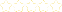• > Crystal Kirch
• > SFHS Math Analysis Summer Review
+# SFHS Math Analysis Summer Review

Owner: Crystal Kirch
##### Description:
These 16 concepts will help you come back to school prepared to succeed in Math Analysis Honors!

### Your Quiz Points in this Playlist

Total Possible
0
16 Tutorials in this Playlist

#### 1. Finding the slope of a line given 2 points

Author: Crystal Kirch
Rating:(12)
This tutorial has a quiz
•• -

#### 2. Writing the equation of a line given slope and a point or two points

Author: Crystal Kirch
Rating:(9)
This tutorial has a quiz
•• -

#### 3. Writing the equations of parallel and perpendicular lines given a line and a point

Author: Crystal Kirch
Rating:(10)
This tutorial has a quiz
•• -

#### 4. Identifying functions while looking at ordered pairs or a graph (vertical line test)

Author: Crystal Kirch
Rating:(8)
This tutorial has a quiz
•• -

#### 5. Evaluating functions with numbers or variable expressions

Author: Crystal Kirch
Rating:(3)
This tutorial has a quiz
•• -

#### 6. Adding and subtracting functions

Author: Crystal Kirch
Rating:(5)
This tutorial has a quiz
•• -

#### 7. Multiplying functions

Author: Crystal Kirch
Rating:(4)
This tutorial has a quiz
•• -

#### 8. Dividing Functions

Author: Crystal Kirch
Rating:(5)
This tutorial has a quiz
•• -

#### 9. Composing functions (including variables and values)

Author: Crystal Kirch
Rating:(3)
This tutorial has a quiz
•• -

#### 10. Finding inverse functions algebraically

Author: Crystal Kirch
Rating:(2)
This tutorial has a quiz
•• -